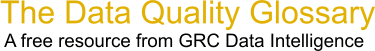Choose Index below for a list of all words and phrases defined in this glossary.

# Slope

index | Index

## Slope - definition(s)

slope - [Euclidean geometry] The incline, or steepness, of a surface. Slope can be measured in degrees from horizontal (0-90), or percent slope (which is the rise divided by the run, multiplied by 100). A slope of 45 degrees equals 100 percent slope. As slope angle approaches vertical (90 degrees), the percent slope approaches infinity. The slope of a TIN face is the steepest downhill slope of a plane defined by the face. The slope for a cell in a raster is the steepest slope of a plane defined by the cell and its eight surrounding neighbors.

[Category=Geospatial ]

Source: esri, 22 August 2012 09:28:23, http://support.esri.com/en/knowledgebase/GISDictionary/term/abbreviationData Quality Glossary.  A free resource from GRC Data Intelligence. For comments, questions or feedback: dqglossary@grcdi.nl